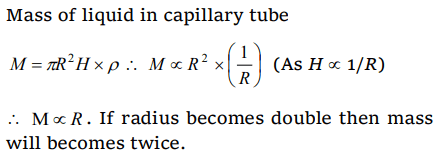## Mechanical Properties of Fluids Questions and Answers Part-17

1. In a capillary tube experiment, a vertical 30 cm long capillary tube is dipped in water. The water rises up to a height of 10cm due to capillary action. If this experiment is conducted in a freely falling elevator, the length of the water column becomes
a) 10 cm
b) 20 cm
c) 30 cm
d) Zero

Explanation: The length of the water column will be equal to full length of capillary tube

2. Radius of a capillary is $2\times 10^{-3} m$   A liquid of weight $6.28\times 10^{-4} N$   may remain in the capillary then the surface tension of liquid will be
a) $5\times 10^{-3} N\diagup m$
b) $5\times 10^{-2} N\diagup m$
c) $5 N\diagup m$
d) $50 N\diagup m$

Explanation: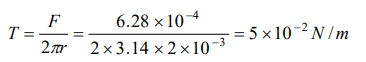3. Two long capillary tubes A and B of radius RB > RA dipped in same liquid. Then
a) Water rise is more in A than B
b) Water rises more in B than A
c) Same water rise in both
d) All of these according to the density of water

Explanation: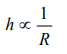4. If water rises in a capillary tube upto 3 cm. What is the diameter of capillary tube ( Surface tension of water = $7.2\times 10^{-2} N\diagup m$    )
a) $9.6\times 10^{-4} m$
b) $9.6\times 10^{-3} m$
c) $9.6\times 10^{-2} m$
d) $9.6\times 10^{-1} m$

Explanation: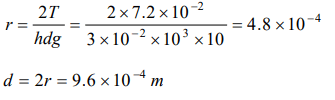5. When a capillary is dipped in water, water rises 0.015 m in it. If the surface tension of water is $7.5\times 10^{-3} N\diagup m$    , the radius of capillary is
a) 0.1 mm
b) 0.5 mm
c) 1 mm
d) 2 mm

Explanation:6. In a capillary tube, water rises to 3 mm. The height of water that will rise in another capillary tube having one-third radius of the first is
a) 1 mm
b) 3 mm
c) 6 mm
d) 9 mm

Explanation: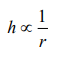7. Kerosene oil rises up the wick in a lantern
a) Due to surface tension of the oil
b) The wick attracts the kerosene oil
c) Of the diffusion of the oil through the wick
d) None of the above

Explanation: Due to surface tension of the oil

8. Water rises against gravity in a capillary tube when its one end is dipped into water because
a) Pressure below the meniscus is less than atmospheric pressure
b) Pressure below the meniscus is more than atmospheric pressure
c) Capillary attracts water
d) Of viscosity

Explanation: Pressure below the meniscus is less than atmospheric pressure

9. A capillary tube of radius R is immersed in water and water rises in it to a height H. Mass of water in the capillary tube is M. If the radius of the tube is doubled, mass of water that will rise in the capillary tube will now be
a) M
b) 2M
c) M/2
d) 4M